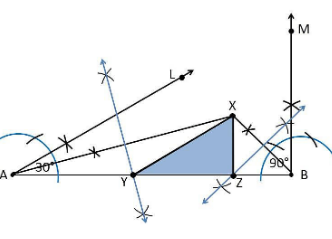Guru

# Construct a triangle XYZ in which ∠Y = 30°, ∠Z = 90° and XY+YZ+ZX = 11 cm. Q.4

• 0

How can i solve this tough question of class 9th ncert of Constructions of math of exercise 11.2  of question no.4. Give me the best and simple way for solving this question. Construct a triangle XYZ in which ∠Y = 30°, ∠Z = 90° and XY+YZ+ZX = 11 cm.

Share

1. Construction Procedure:

The steps to draw the triangle of given measurement is as follows:

1. Draw a line segment AB which is equal to XY+YZ+ZX = 11 cm.

2. Make an angle ∠Y = 30° from the point A and the angle be∠LAB

3. Make an angle ∠Z = 90° from the point B and the angle be ∠MAB

4. Bisect ∠LAB and ∠MAB at the point X.

5. Now take the perpendicular bisector of the line XA and XB and the intersection point be Y and Z, respectively.

6. Join XY and XZ

7. Therefore, XYZ is the required triangle• 0